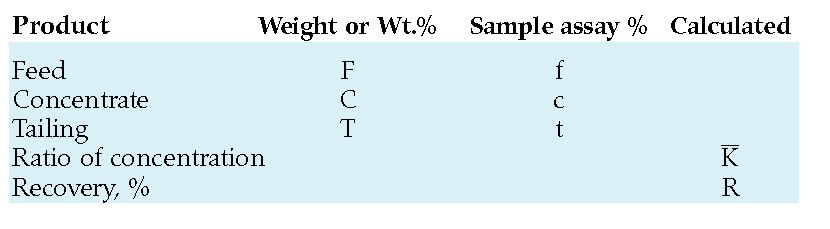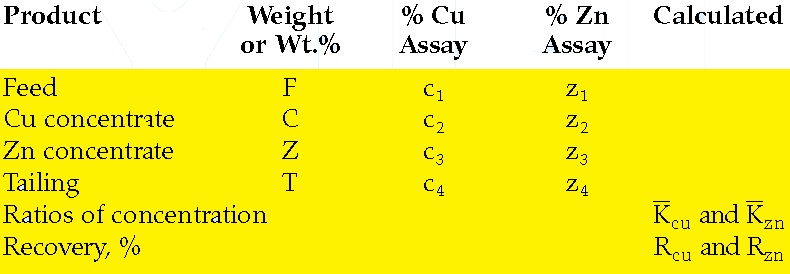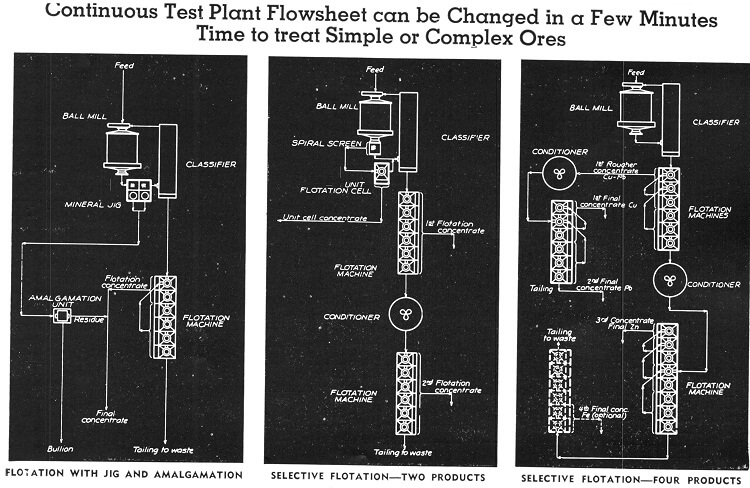# Metallurgical Accounting Formulas | Concentration and Recovery in Mineral Processing

Have you been looking for a quick way how to calculate your flotation circuit’s metal recovery?  You have no concentrate weights all you have are metal assays.  Here are the assay based recovery formulas you can use for your metallurgical accounting.  Using these formulas, the Metallurgical/Metallurgy performance of the concentration plant or of a particular mill circuit is readily assessed.  They are similarly applied for calculating the results of laboratory testing.  Since the computations are entirely dependent on the assays and weights, where known, of the process feed and products of separation, the calculated results are only as accurate as the sampling, assaying, and weighing methods employed to obtain the required data. As will also be seen, any increase in the number of separations and mineral components to be accounted for, greatly increases the complexity of the computations.

### Two Product Formula Online Calculator <– Click to Use

Applicable to the simplest separation where only one concentrate and one tailing result from a given ore feed.Ratio of concentration can be thought of as the number of tons of feed required to produce 1 ton of concentrate. The ratio, K, for a
separation can be obtained directly from the product weights or from the product assays if the weights are not known:

K = F /C  = (c — t)/(f-t) = the concentration ratio

At operating plants, it is usually simpler to report the K based on assays. If more than one mineral or metal is recovered in a bulk concentrate, each will have its own K with the one regarded as most important being reported as the plant criteria. If the tonnage of concentrates produced is unknown it can be obtained using the product assays and the tons of plant feed:

C = F/K = F  (f — t) / (c-t) = the weight of the concentrate.

Recovery %

Represents the ratio of the weight of metal or mineral value recovered in the concentrate to 100% of the same constituent in the heads or feed to the process, expressed as a percentage. It may be calculated in several different ways, depending on the data available.

By assays f, c and t only:

• R = 100 c(f — t)/f (c — t)  = recovery %
• By K plus assays f and c
• R = 100 c/Kf = recovery %
• By weights F and C, plus assays c and t
• R = 100 Cc / (Cc+t(F—C)) = recovery %

A copper concentrator is milling 15,000 tons/day of a chalcopyrite ore assaying 1.15% copper. The concentrate and tailings produced average 32.7% and 0.18% copper, respectively.  I let you do the math!

Three product (bi-metallic) formulas

Frequently, a concentrator will mill a complex ore requiring the production of two separate concentrates, each of which is enriched in a different metal or valuable mineral, plus a final tailing acceptably low in both constituents. Formulas have been developed which use the feed tonnage and assays of the two recovered values to obtain the ratios of concentration, the weights of the three products of separation, and the recoveries of the values in their respective concentrates. For illustrative purposes data from a copper-zinc separation is assumed.The ratios of concentration, Kcu and Kzn are those for the copper and zinc concentrates, respectively, with Rcu and Rzn the  percentage recoveries of the metals in their corresponding concentrates. As follows:

• C = F (c1 — c4)(z3 — z4) — (z1 — z4)(c3 — c4) / (c2 — c4)(z3 — z4) — (z2 — z4)(c3 — c4) = tons Cu concentrate
• Z = F  (c2 — c4)(z1 — z4) — (c1 — c4)(z2 — z4) /  (c2 — c4)(z3 — z4) — (z2 — z4)(c3 — c4) = tons Zn concentrate
• Rcu = 100 C x c2 / F x c1   = copper recovery %
• Rzn = 100 Z x z3 / F x z1 = zinc recovery %
• Kcu = F/C and Kzn = F/Z = ratio of concentration

The three product solution illustrated above can be somewhat simplified by taking an intermediate tailings sample between the two stages of concentration; i.e., a copper tail (zinc feed) sample in the previous example.

Copper tail (zinc feed) = CT with copper and zinc assays = c 5 and z5

Assume mill feed, F, as Unity 1
Then, C + CT = 1
(C x c2) + (CT x c5) = c1
(C x c5) + (CT x c5) = c5

Subtracting both above,
C(c2 — c5) = (c1 — c5)

Then, C = F (c1 — c5)/(c2 — c5) = tons copper concentrate  and similarly, Z = (F — C)  (z5 — z4) / (z3 — z4)= tons zinc concentrate.

It is decided to take a copper tail (zinc feed) sample in order to provide a check on the results calculated in the previous example. The sample (CT) assayed 0.55% Cu (c5) and 20.9% Zn (z5), respectively. The check weights of the copper and zinc concentrates are computed using the above math.